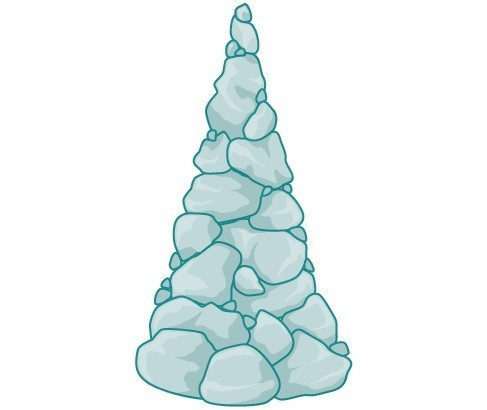## Introduction to Inverses and Radical Functions

### LEARNING OBJECTIVES

By the end of this lesson, you will be able to:

• Find the inverse of a polynomial function.
• Restrict the domain to find the inverse of a polynomial function.Figure 1

A mound of gravel is in the shape of a cone with the height equal to twice the radius.

The volume is found using a formula from elementary geometry.

$\begin{cases}V=\frac{1}{3}\pi {r}^{2}h\hfill \\ \text{ }=\frac{1}{3}\pi {r}^{2}\left(2r\right)\hfill \\ \text{ }=\frac{2}{3}\pi {r}^{3}\hfill \end{cases}\\$

We have written the volume V in terms of the radius r. However, in some cases, we may start out with the volume and want to find the radius. For example: A customer purchases 100 cubic feet of gravel to construct a cone shape mound with a height twice the radius. What are the radius and height of the new cone? To answer this question, we use the formula

$r=\sqrt{\frac{3V}{2\pi }\\}$

This function is the inverse of the formula for V in terms of r.

In this section, we will explore the inverses of polynomial and rational functions and in particular the radical functions we encounter in the process.